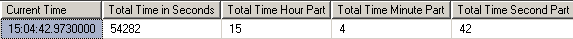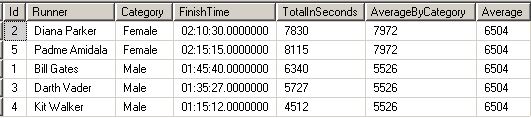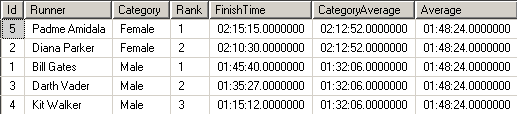﻿ How to Calculate Time Operations in SQL Server on Time Data TypeSQL Server and T-SQL Development Tutorials Development resources, articles, tutorials, code samples, tools and downloads for ASP.Net, SQL Server, Reporting Services, T-SQL, Windows, AWS, SAP HANA and ABAP

# How to Calculate Time Operations in SQL Server on Time Data Type

It is sometimes necessary to deal with time variables, take average of time columns or a group of rows including time fields. Frequently, t-sql developers sum time fields and then return total time variable as and expression in hour, minute and seconds.

To complete such a time operation task, I generally choose to convert time variable into seconds. Then after completing mathematical operations over time values expressed in seconds, I can return back the final result as a time variable. It is easy to convert time expressed in seconds into a time variable with hours, minutes and seconds part in it.

This T-SQL tutorial will be showing how to convert time into seconds and then covert it back to time variable from pure seconds expression.

The following SQL script gets the time part of GetDate() function into a SQL Server time variable @t.
The time value @t is expressed as a time period in seconds. This requires the following calculation.
Multiply hours with 3600 seconds/hour
Multiply minutes with 60 seconds/hour
Add hour-to-seconds, minutes-to-seconds and remaining seconds part into resultant @total integer variable.
select @total = DATEPART(ss,@t) + 60 * DATEPART(mi,@t) + 3600 * DATEPART(hh,@t)

The second SELECT statement re-calculates the time parts (hour, minute, second) of the @total time in seconds.
This sql calculation is simple.
Divide @total period expressed in seconds into 3600. This returns hours
Take MOD to 3600 of @total time period in seconds. Then divide resultant value into 60 for minutes
Take MOD to 60 of @total time for seconds.

SQL developers can use the above logic to express a time span which is expressed in seconds as a time variable with hour, minute and seconds part.

Here is the output of the above t-sql script.## Convert Time Variable into Seconds

Here is a user-defined SQL function that programmers can use to convert time value into seconds. I'll use this function in the following sql codes within this t-sql tutorial.

## Sample Table Data with Time Column

Now let's set up a SQL problem. Assume that you simulate the calculation of marathon running results. Here is a database table where you keep results of the marathon with finish time in SQL time variable. The CREATE TABLE command is followed by sample data generating INSERT statement.

Now we can calculate average results in each runner category and overall average simply using SQL AVG() aggregate function over finish time converted into seconds using the user-defined function fn_CalculateTimeInSeconds

Please note that the SQL AVG() function enhancement with the OVER clause. The Partition By clause is grouping the results into categories. And a dummy "Partition By 1" clause returns an overall Average calculation over all result set.## Convert Time in Seconds into Time Variable

Now SQL developers need a function which will convert the integer seconds value into a time data type value.
Check the following user function fn_CreateTimeFromSeconds SQL code. You will realize that the division and mod operations are familiar from the tutorial entry section.
The only different part is string formatting with '00' concatenation and Right() string function.
This sql codes are necessary to format the results in HH:MI:SS format.

## Time Calculation in SQL Server

Here is the T-SQL CTE expression which makes a time calculation on sample data and returns the results again in SQL Server time data type.

Please note that the below SQL CTE expression runs the three steps required for a SQL time calculation in order:
Convert time into seconds,
Make aritmetic operations using SQL functions,
Convert result into SQL Server time data type.

CTE 1 uses user function fn_CalculateTimeInSeconds() to convert time column FinishTime into seconds with data type integer.

CTE 2 performs SQL arithmetic calculations like AVG() average aggregation function

Last SELECT returns SQL calculation results in SQL Server Time data type using function fn_CreateTimeFromSeconds()SQL Server